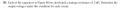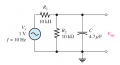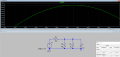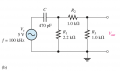# Thevenizing a leaky capacitor

#### SamR

Joined Mar 19, 2019
4,811
Been puzzling over this for a few days. I modeled it in LTS to verify the Vo and it's not what I'm calculating.Thevenized you have 3 parallel resistors, 10k, 10k, 2k = 1429Ω = Rth
Vth = (Rleak/((R1||R2)+Rleak)Vs = 285.7mV ? Or Vth = ?
Xc = 3388Ω
.: Vo = ((Xc/√Rth^2+Xc^2)Vth = 263.24mV

However, from LTS for the Vo I get 132mV#### AlbertHall

Joined Jun 4, 2014
12,267

#### SamR

Joined Mar 19, 2019
4,811
Thevenized they are and as a voltage divider it is as if the series 10k is only 5k because of the other 10k resistor.

#### AlbertHall

Joined Jun 4, 2014
12,267
In the Vth calculation, which resistors are in parallel?

#### SamR

Joined Mar 19, 2019
4,811
R1, R2, Rleak are all parallel Thevenized. That is where I am confused. With only R1 and Rleak I have no problem. The placement of R2 added into the mix is where I am having a problem. So I used R1||R2. Should I instead look at it (R2||Rleak/(R1 + R2||Rleak))Vs? Nope, that gave me 400mV.

#### SamR

Joined Mar 19, 2019
4,811
I also tried (Xc/√(Rth^2 + Xc^2))Vs

#### WBahn

Joined Mar 31, 2012
29,154
Been puzzling over this for a few days. I modeled it in LTS to verify the Vo and it's not what I'm calculating.
View attachment 202057
View attachment 202058

Thevenized you have 3 parallel resistors, 10k, 10k, 2k = 1429Ω = Rth
Vth = (Rleak/((R1||R2)+Rleak)Vs = 285.7mV ? Or Vth = ?
Xc = 3388Ω
.: Vo = ((Xc/√Rth^2+Xc^2)Vth = 263.24mV

However, from LTS for the Vo I get 132mV
Let's ask some simple sanity questions.

If you are including the leakage resistance as part of the Thevenin equivalent (which it appears you are doing) then you should get a resistance of significantly less than 2 kΩ but no less than 1 kΩ, which you did. So that's reasonable.

The Thevenin voltage is then going to be a voltage divider with something between 1 kΩ and 2 Ω at the bottom (10 Ω || 2 kΩ) and 10 Ω at the top. The LEAST and MOST this can be would be

Vth_min = (1 V)(1 kΩ)/(1 kΩ + 10 kΩ) = 90.9 mV
Vth_max = (1 V)(2 kΩ)/(2 kΩ + 10 kΩ) = 167 mV

When you are calculating Vth, R1 and R2 are NOT in parallel because they do not have the voltage applied across them. When finding Rth they are in parallel because you have turned V2 to 0 V which means that the two resistors DO have the same voltage applied to them.

#### SamR

Joined Mar 19, 2019
4,811
OK so Rload = R2||Rleak = 1667Ω then the voltage divider becomes R1 & Rload

Vo = ((Xc/√(Xc^2 + Rth^2))Vth = 132mV BINGO! Thanks!

EDIT: Ah nooo... The LTS is Vp, not Vrms... arghhh...

Last edited:

#### SamR

Joined Mar 19, 2019
4,811
In the second part of the question, they use an RC lead circuit.Xc = 3388Ω EDIT: Egad! Xc = 1/6.28*100kHz*470pF = 7.48E-6Ω See next post
if I remove the C and Thevenize Rth = R3||(R1+R2) = 762Ω
now to find Vth, I want to R1|| a voltage divider of R2 & R3? At this point I am lost.

I tried to simplify it with just C and R1 as Vo = (Xc/Z)Vs = 4.19V EDIT: (R1/Z)Vs = 2.7V and feed that into the R2 & R3 voltage divider to get a Vo = 2.1V 1.36V but that is not what I am getting from LTS

Here across R1 is 1.47 Vo across R3 is 735mVLast edited:

#### WBahn

Joined Mar 31, 2012
29,154
In the second part of the question, they use an RC lead circuit.

View attachment 202109

Xc = 3388Ω EDIT: Egad! Xc = 1/6.28*100kHz*470pF = 7.48E-6Ω See next post
if I remove the C and Thevenize Rth = R3||(R1+R2) = 762Ω
now to find Vth, I want to R1|| a voltage divider of R2 & R3? At this point I am lost.

I tried to simplify it with just C and R1 as Vo = (Xc/Z)Vs = 4.19V EDIT: (R1/Z)Vs = 2.7V and feed that into the R2 & R3 voltage divider to get a Vo = 2.1V 1.36V but that is not what I am getting from LTS

Here across R1 is 1.47 Vo across R3 is 735mV
Let's again do some sanity check estimation before getting into the details. Imagine R1 was 2.0 kΩ instead of 2.2 kΩ. Then the combination of R1, R2, and R3 (let's call it Ro) would be 1.0 kΩ in series with C and because R2 and R3 form a 50% voltage divider, whatever voltage appears across Ro, the value of Vo will be half of that.

So let's look at two extremes. In the first case, the impedance of the capacitor is dominated by the leakage resistance, Rc, of 2 kΩ. That would make Ro and Rc form a voltage divider making the voltage across Ro 1/3 of Vs and Vo being 1/2 of that, or 1/6 of 5 V, which is 833 mV. That would be the case at low frequencies, such as DC (with the caveat that we are estimating R1 to be 10% smaller than it really is). As the frequency increases, the Xc of the capacitor goes down and at sufficiently high frequencies it shorts out Rc and makes the output close to 1/2 of Vs, or about 2.5 V. So our answer should be somewhere between those values (again, subject to the caveat mentioned previously).

So what is the Xc of the capacitor at 100 kHz?

Xc = -1/(ωC) = -1/( (2*3.14156 radians/cycle) * (100 kcycles / s) * (470 pF) ) = -3.39 kΩ

So the capacitor is more resistive than reactive, but the reactive part is small enough that it can't be ignored.

One recommendation I would make is to take some time to learn about how to work with complex numbers and then complex impedances. It really will make all of this so much easier.

#### SamR

Joined Mar 19, 2019
4,811
Xc = -1/(ωC) = -1/( (2*3.14156 radians/cycle) * (100 kcycles / s) * (470 pF) ) = -3.39 kΩ
I am getting 7.43E-6 for this?

One recommendation I would make is to take some time to learn about how to work with complex numbers
That I know how to do but not sure how it applies here? At this point in Floyd's Electronic Fundamentals, complex numbers have not been brought in as yet.

and then complex impedances
Still on my to-do list is Malvino's Resistive and Reactive Circuits.

I think I do not completely understand the reactive effect on ALL of the elements of the circuit. I broke the circuit down in the previous solution but in the last branch, there is no reactive element in the solution? Is that the correct solution?

#### The Electrician

Joined Oct 9, 2007
2,936
I am getting 7.43E-6 for this?
WBahn has appropriate parentheses in his expression.

You have: "Xc = 3388Ω EDIT: Egad! Xc = 1/6.28*100kHz*470pF = 7.48E-6Ω "

If you would have one pair of parentheses like this: Xc = 1/(6.28*100kHz*470pF)

you would get the correct result.

Also, you aren't properly handling the situation where a voltage divider is followed by another voltage divider. The action of the first voltage divider is affected by the loading effect of the second voltage divider, and you haven't taken that into account,

•SamR

#### SamR

Joined Mar 19, 2019
4,811
Yes, I had the parenthesis correct in my calculation. I should have typed it as 1/(6.28*100kHz*470pF)

I was questioning the lack of reactive effect on the 2nd voltage divider... Back to the books...

#### The Electrician

Joined Oct 9, 2007
2,936
Due to the very low resistive value of Xc, it pretty much washes out in the calculations for V at Rt.
View attachment 202189
Shouldn't you replace the incorrect value 7.43E-6Ω with the correct value? Also, what about the 2k leakage resistance in parallel with the capacitance?

#### SamR

Joined Mar 19, 2019
4,811
Shouldn't you replace the incorrect value 7.43E-6Ω with the correct value? Also, what about the 2k leakage resistance in parallel with the capacitance?
1/(6.28*100000Hz*470pF) = 7.42E-6Ω

Arghhh. Yes, I forgot Rleak. Need to wake up still...

#### WBahn

Joined Mar 31, 2012
29,154
2.5 V is a reasonable PROVIDED the impedance of the capacitor is in the microohm range. But is that in any way a reasonable impedance for a 470 pF capacitor at 100 kHz?

For comparison, one inch of #24 hookup wire has about 200 μΩ of resistance.

We know that the impedance of a capacitor goes UP as the capacitance goes down and 470 pF (less than half a nanofarad) is a very small capacitor.

We know that the impedance of a capacitor goes UP as the frequency goes down and 100 kHz is actually a pretty low frequency.

So we would expect a rather large reactance for a capacitor of that size at that frequency.

Now let's look at an approximate answer.

Xc = 1/(6.28*100kHz*470pF)

In the denominator we have something like 5 * 100 * 500 = 250000 = 0.25*10^6 for the coefficients and 10^3 * 10^-12 = 10^-9 for the scaling factors. So that gives us about 0.25 * 10^-3

When we take the recipricol we have 1/0.25 which is 4 multiplied by 10^3, so we expect something in the 4000 Ω range.

If your calculation ends up significantly different than that (more than a factor of two or so), then you need to step back and resolve it. Either your calculation was wrong or your estimate was wrong. Don't go forward until they are in agreement.

#### WBahn

Joined Mar 31, 2012
29,154
1/(6.28*100000Hz*470pF) = 7.42E-6Ω

Arghhh. Yes, I forgot Rleak. Need to wake up still...
Xc is the REACTANCE of the capacitor. It does not include the resistive component. That is not the problem.

How are you getting 7.42E-6Ω ?

You certainly aren't getting it by carefully tracking your units!

As best I can tell, you are getting it by going:

$$\left( \frac{1}{6.28} \right) \left( 100000 \right) \left( 470E-12 \right)$$

getting 7.48E-6 and then tacking on Ω because that is the unit that you expect, want, and pray that the answer happens to have.

TRACK THE UNITS!!!!

If you had done that, you would discover that your answer has units of (cycles^2 per ohm) when you need it to have units of ohms.